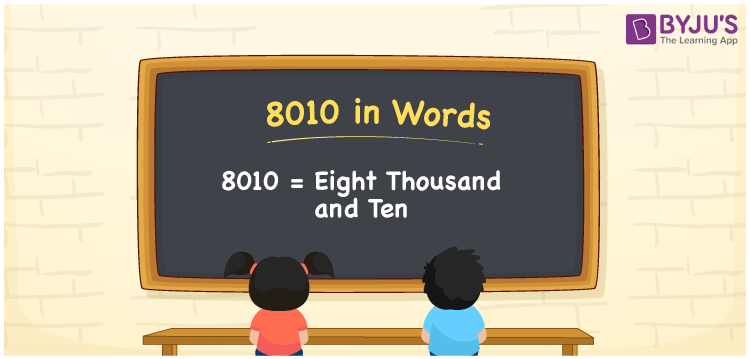# 8010 in Words

We can write 8010 in words as Eight thousand and ten. Suppose you purchased a backpack that costs Rs. 8010, you can say, “I purchased a backpack worth Eight thousand and ten rupees”. We can derive the number 8010 into words with the help of a place value chart. Let’s learn how to write the cardinal number 8010 in word form here, in this article.

 8010 in words Eight thousand and ten Eight thousand and ten in Numbers 8010

## 8010 in English Words

We generally express numbers in words using the English alphabet. Thus, we can write 8010 in English as “Eight thousand and ten”.## How to Write 8010 in Words?

The conversion of 8010 into words is simple when we use the place value chart. This can be done as follows.

 Thousands Hundreds Tens Ones 8 0 1 0

Here, ones = 0, tens = 1, hundreds = 0, thousands = 8

Now, expand these digits with respect to their place values defined above.

8 × Thousand + 0 × Hundred + 1 × Ten + 0 × One

= 8 × 1000 + 0 × 100 + 1 × 10 + 0 × 1

= 8000 + 10

= Eight thousand + Ten

= Eight thousand and ten

Therefore, 8010 in words = Eight thousand and ten.

Also, check: place value

As we know, 8010 is a natural number that precedes 8011 and succeeds 8009.

8010 in words – Eight thousand and ten

Is 8010 an odd number? – No

Is 8010 an even number? – Yes

Is 8010 a perfect square number? – No

Is 8010 a perfect cube number? – No

Is 8010 a prime number? – No

Is 8010 a composite number? – Yes

## Frequently Asked Questions on 8010 in Words

Q1

### How do you write 8010 in words?

We can write the number 8010 in words as Eight thousand and ten.
Q2

### How to write an amount of Rs. 8010 in words?

We can write an amount of Rs. 8010 in words as “Eight thousand and ten rupees”.
Q3

### Express the value of 8010 – 1000 in words.

8010 – 1000 = 7010 Thus, we can express the value of 8010 – 1000, i.e. 7010 in words as Seven thousand and ten.